# Ncert Maths solutions for Class 6-chapter 7 exercise 7.4 Fractions

Solutions for Class 6 Ncert Maths chapter 7 Fractions exercise 7.4 are given.

First you should study the textbook lesson Fractions very well.

You should observe the example problems and solutions given in the textbook. Try them well.

Observe the given below solutions and try them in your own method.

You can see.

NCERT maths solutions for class 6

Fractions

Exercise 7.1

Exercise 7.2

Exercise 7.3

Exercise 7.4

Exercise 7.5

Exercise 7.6

Class 6 Chapter 7 Fractions exercise 7.4 solutions Ncert

Chapter – 7

Fractions

Exercise 7.4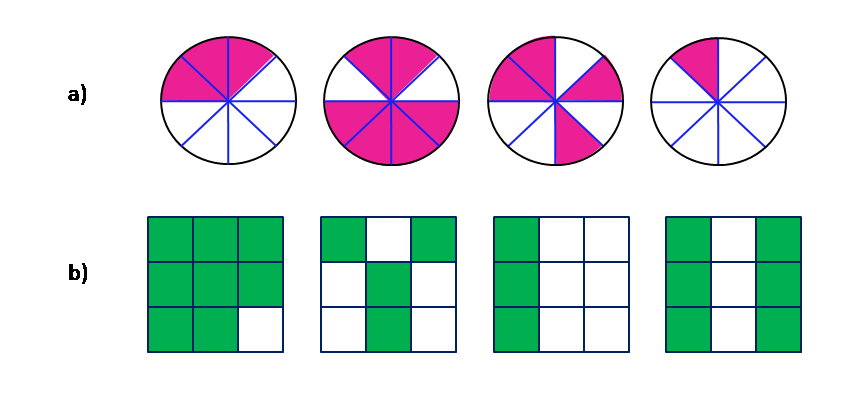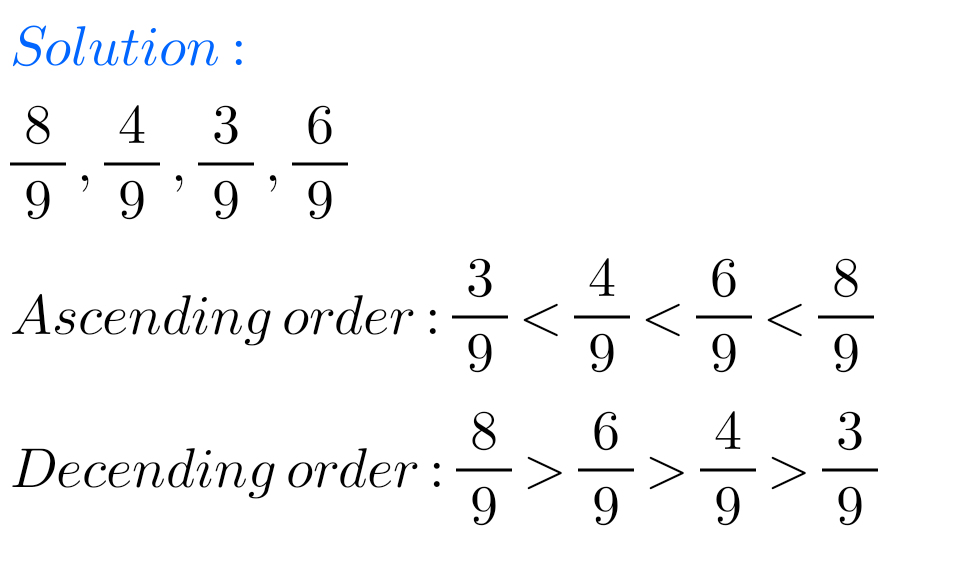F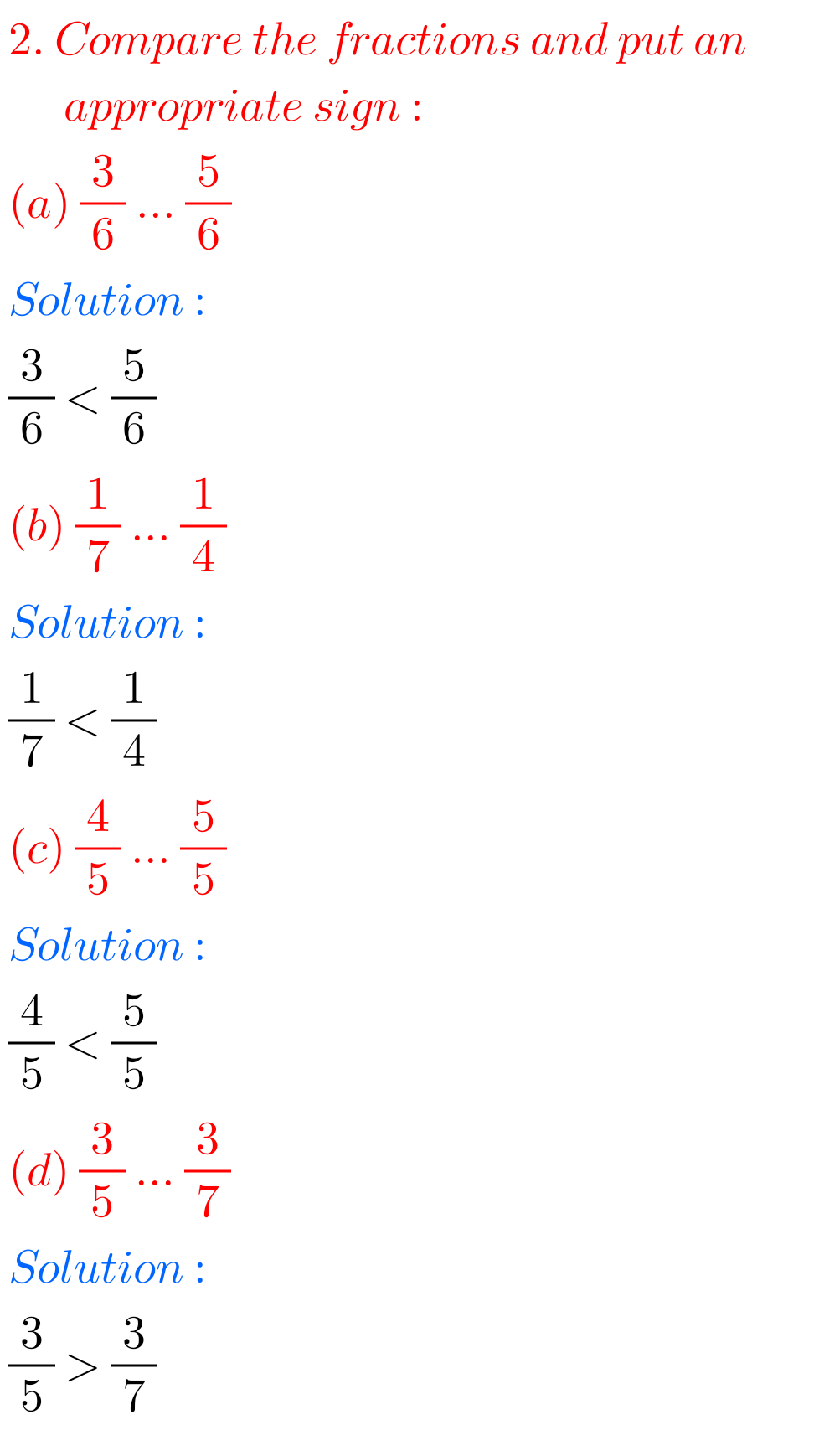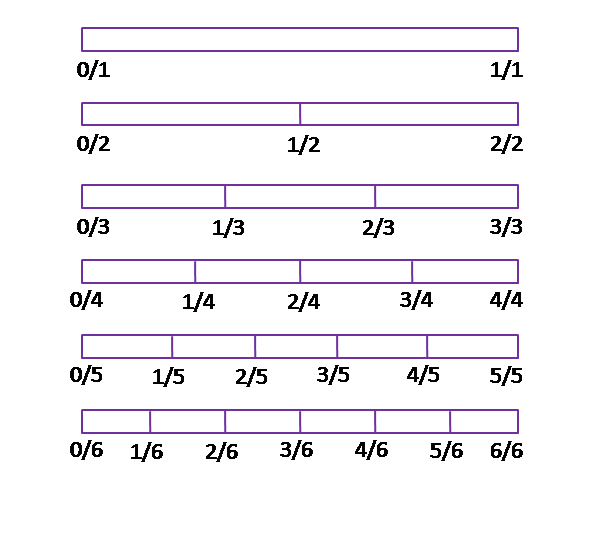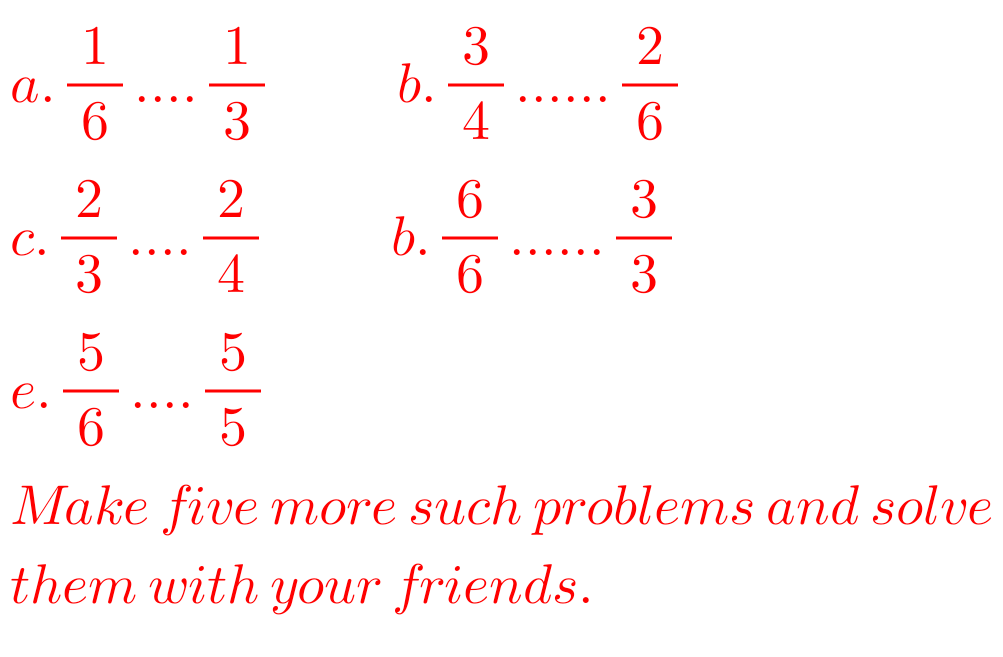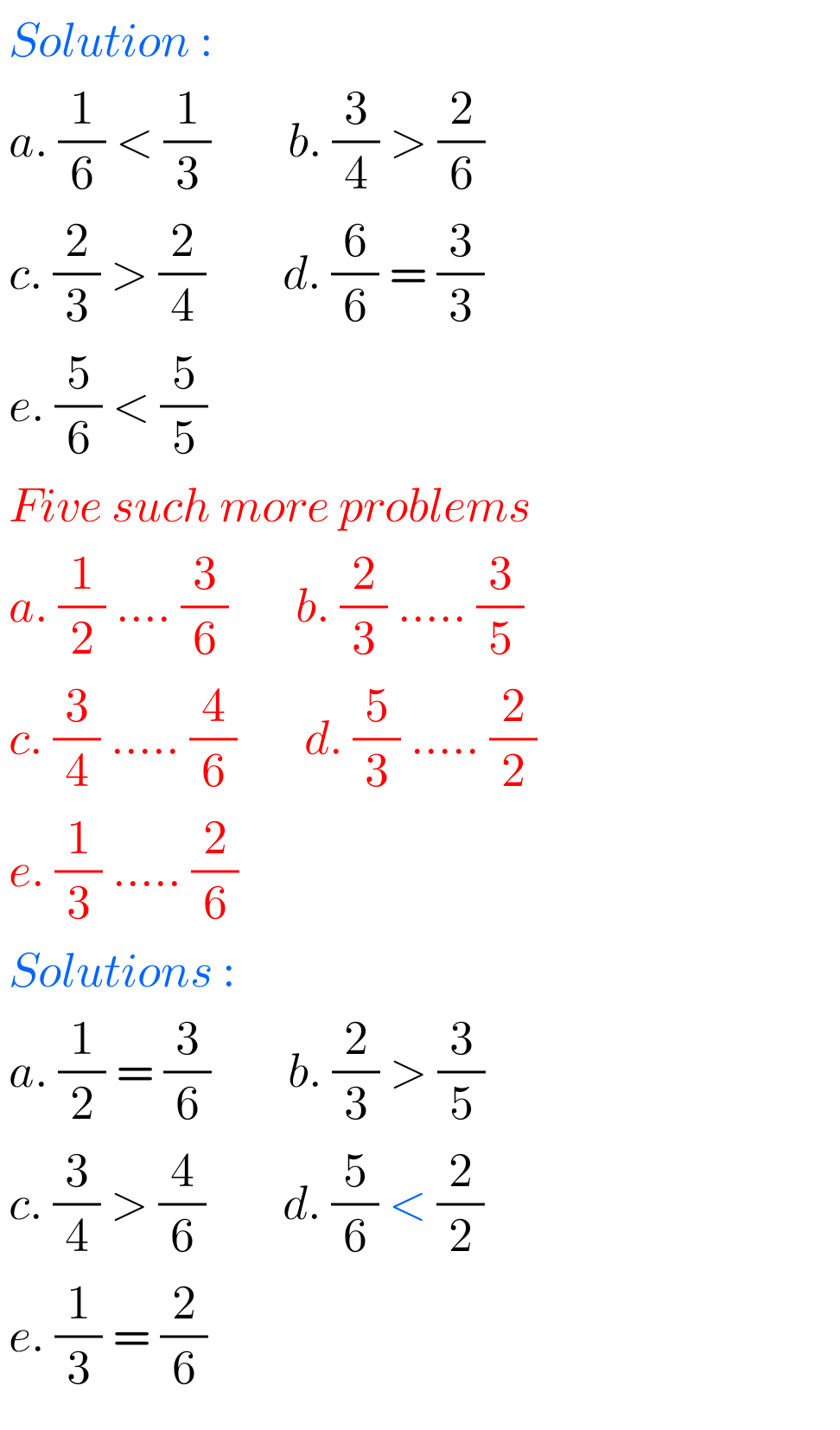#### Exercise 7.4 Fractions class 6 solutions Ncert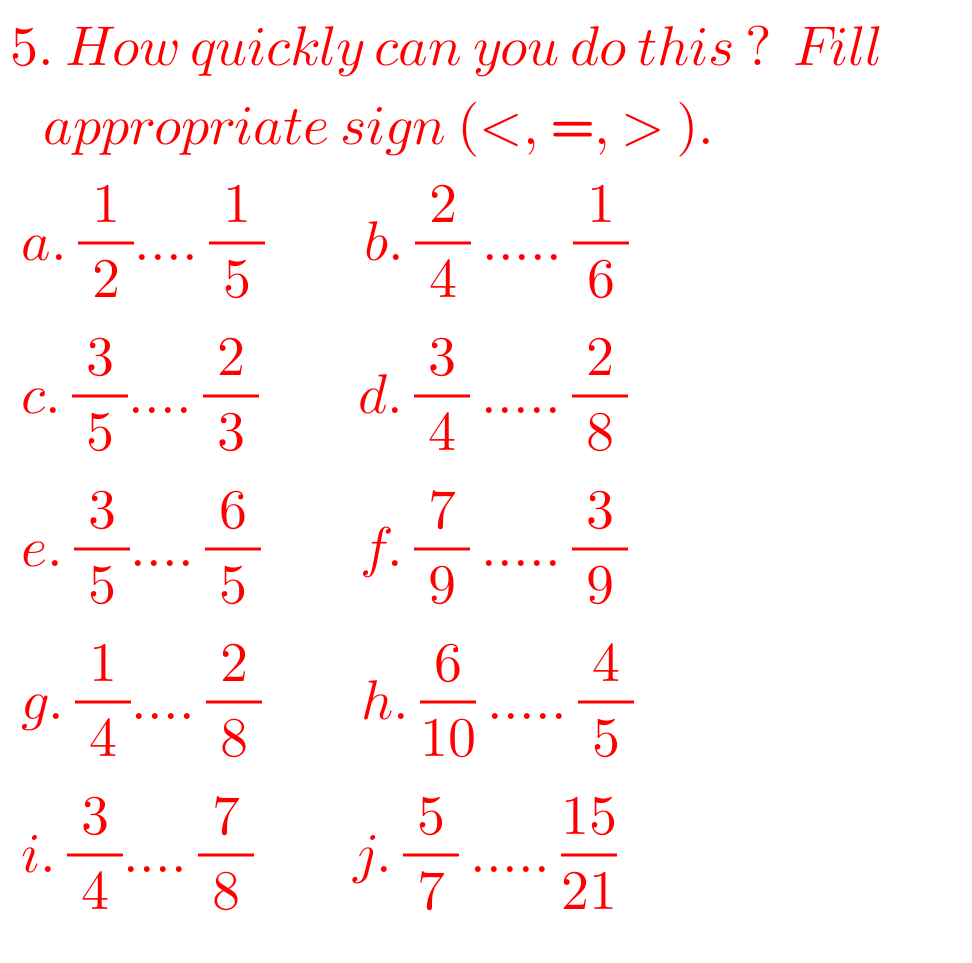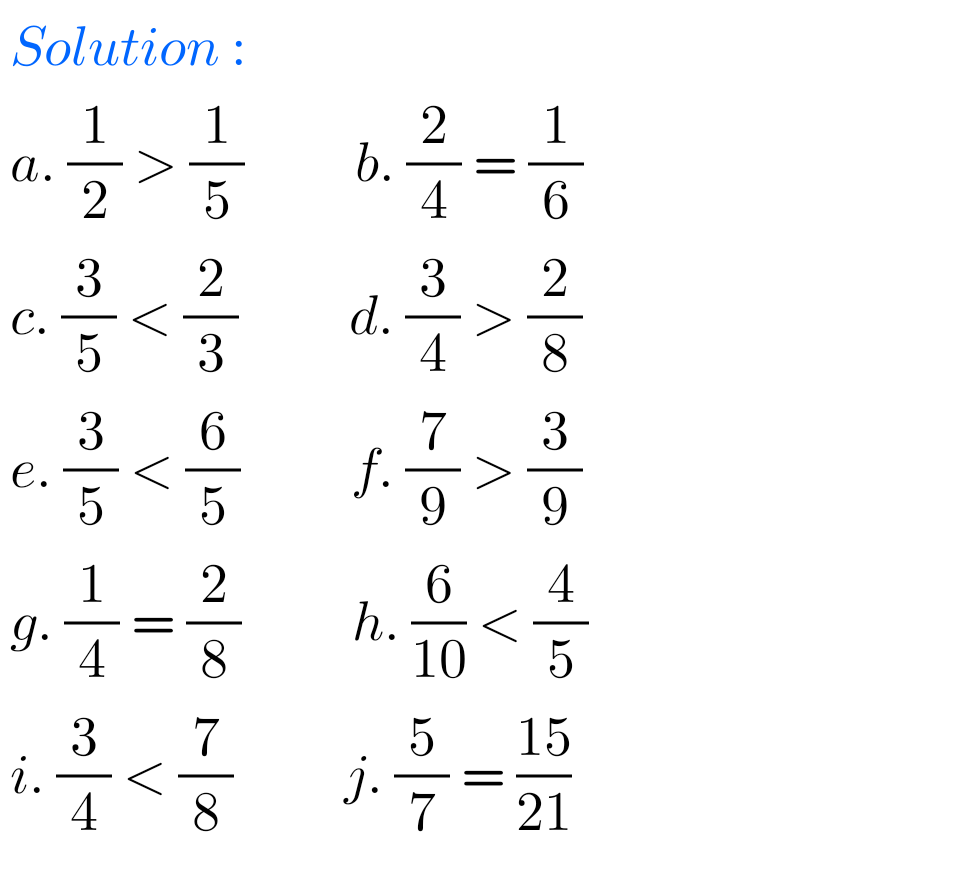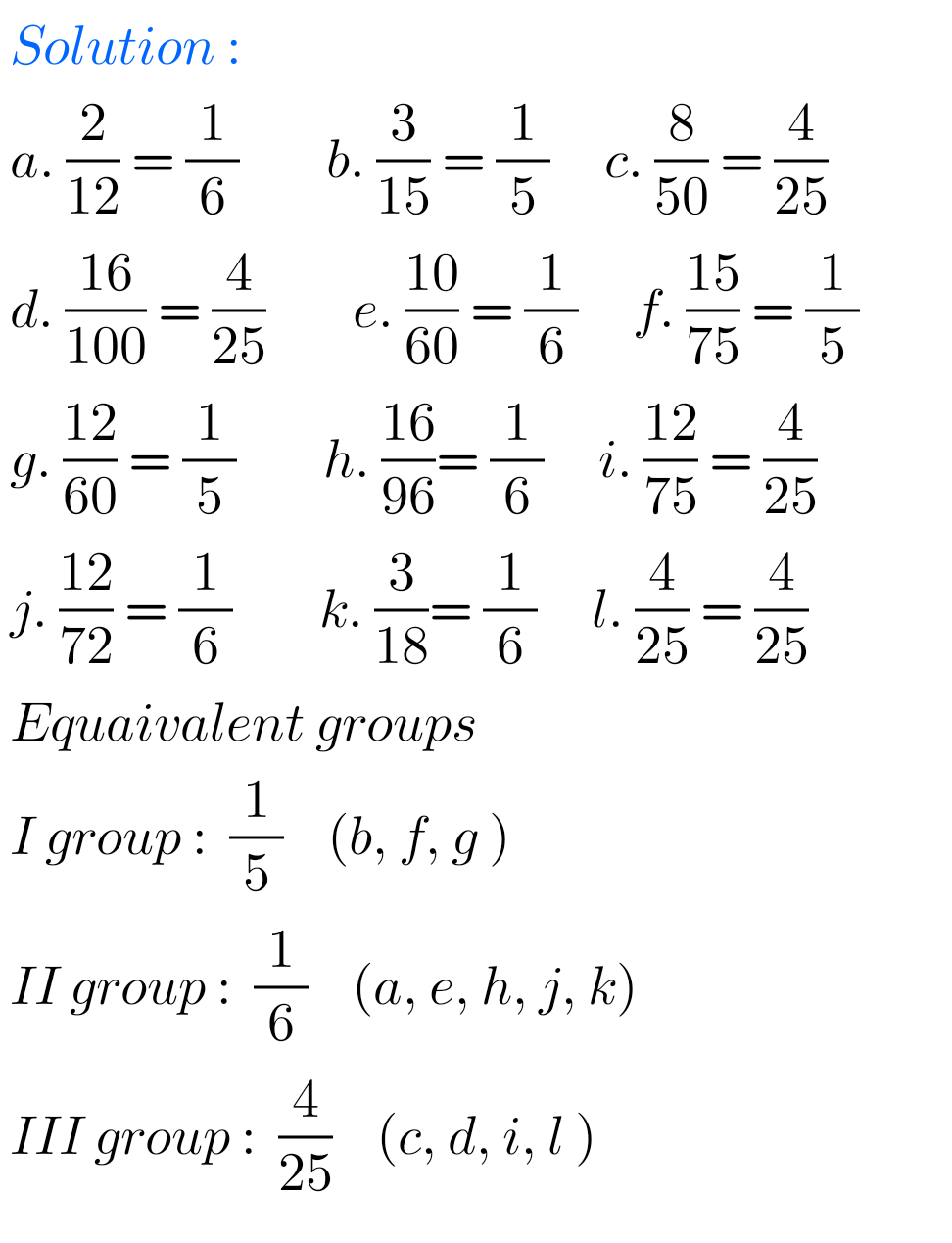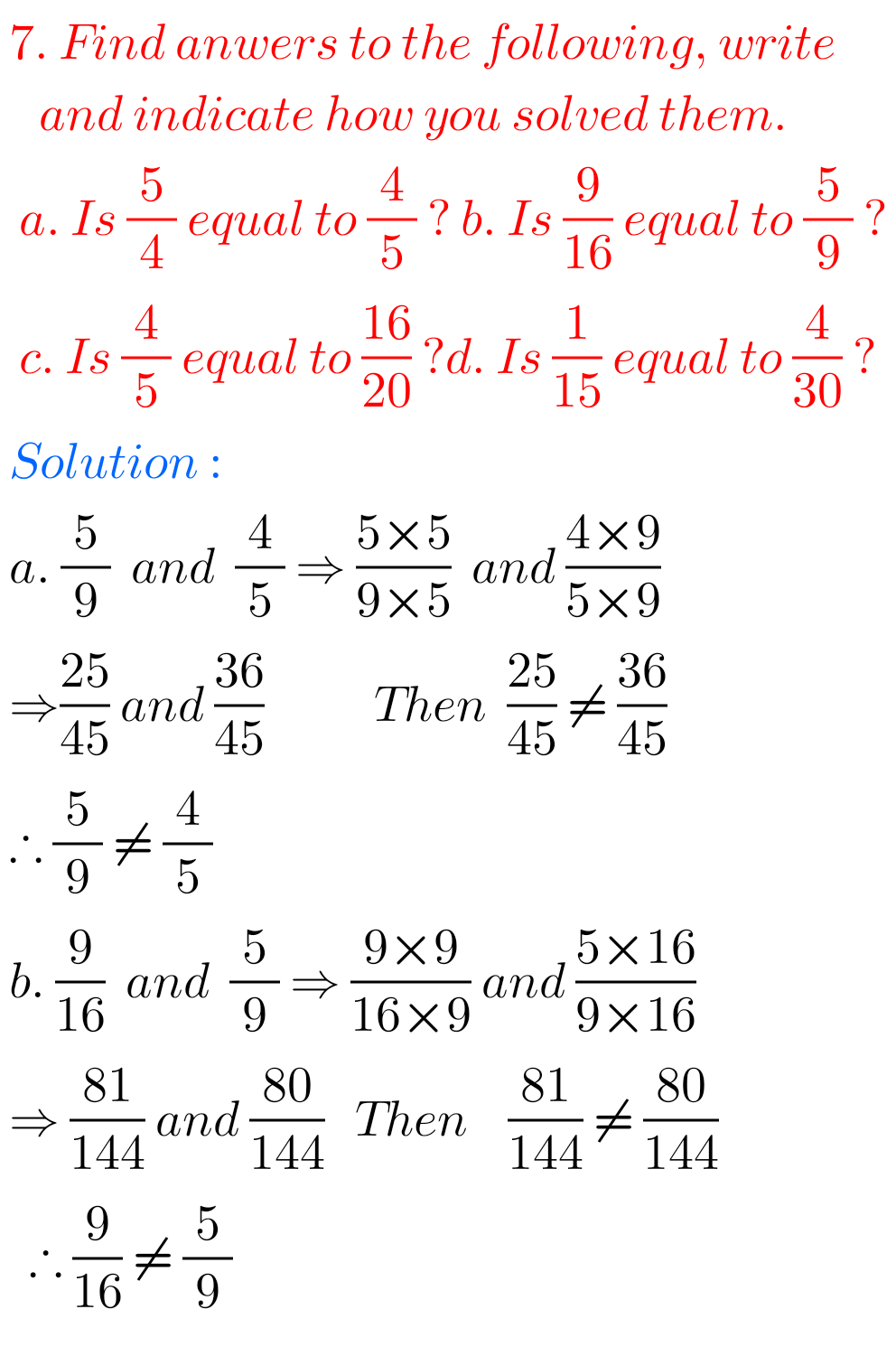##### Solutions for Fractions exercise 7.4 class 6th ncertNote: Observe the solutions and try them in your own method.

NIOS Maths 311 book 2 Solutions for some chapters

NCERT class 6 maths solutions

NCERT class 7 maths solutions

Inter maths solutions

SSC maths Solutions class 10